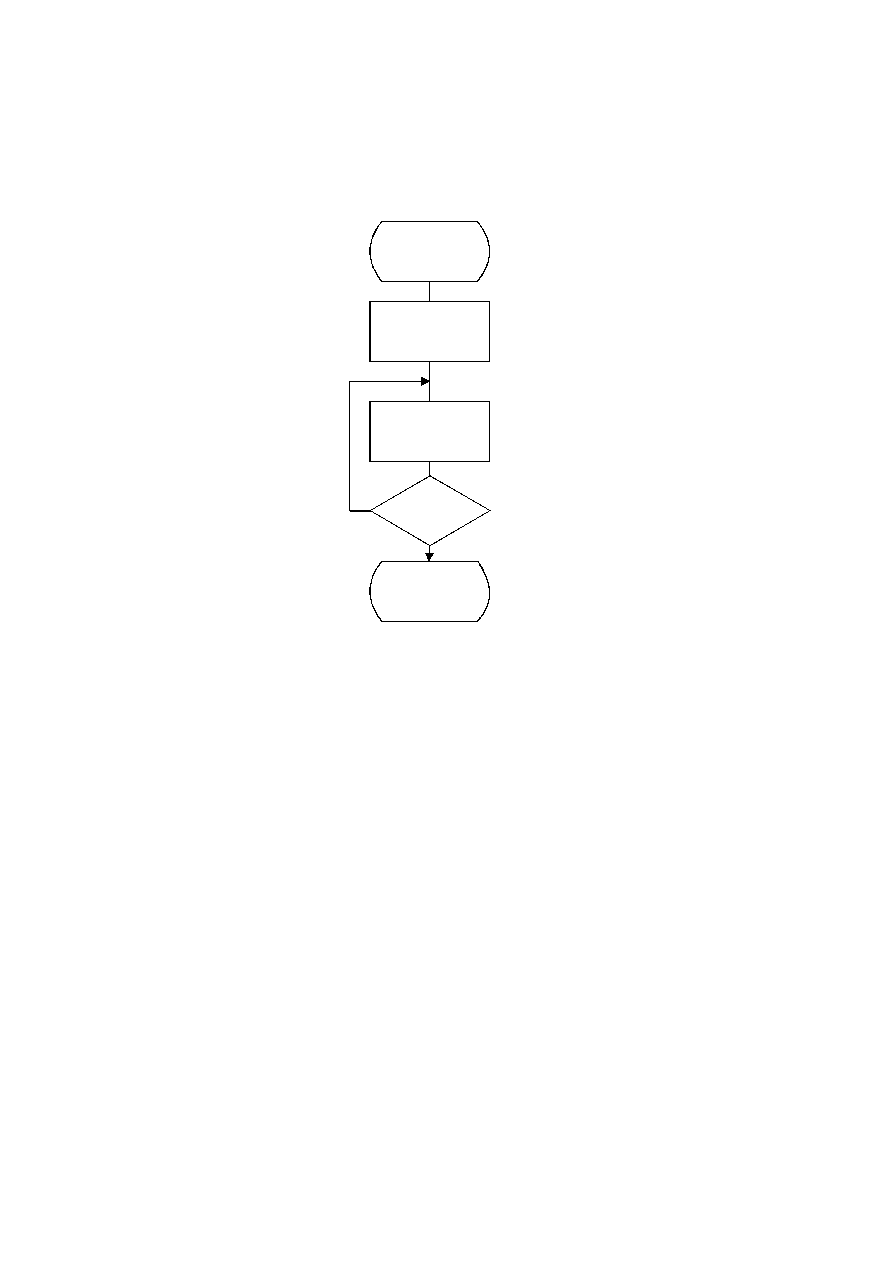ISO/IEC 10918-1 : 1993(E)
E.2.5
Control procedure for decoding a minimum coded unit (MCU)
The procedure for decoding a minimum coded unit (MCU) is shown in Figure E.10.
In Figure E.10 Nb is the number of data units in a MCU.
The procedures for decoding a data unit are specified in Annexes F, G, and H.
TISO1320-93/d070
Decode_MCU
N = 0
Done
Yes
No
N = N + 1
Decode_data_unit
N = Nb
?
Figure E.10 ­ Control procedure for decoding a minimum coded unit (MCU)
Figure E.106 [D70], = 11.5 cm = 449.%
86
CCITT Rec. T.81 (1992 E)